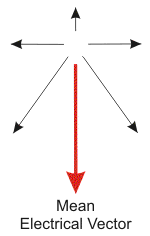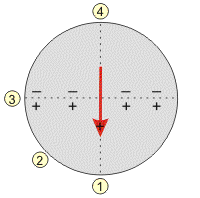# Mean Electrical Vector

When the atria (or ventricles) undergo depolarization, the wave of depolarization that spreads across the muscle mass occurs in many directions simultaneously. If a snapshot of electrical activity could be taken at an instant in time during the process of depolarization, many individual waves of depolarization represented by arrows could be observed (black arrows in below figure). Each arrow represents a different individual vector representing depolarization. The mean electrical vector for all the individual vectors is also shown in the figure (red arrow). This mean electrical vector represents the sum of all of the individual vectors at a given instant in time.It is this instantaneous mean electrical vector that is "seen" by the recording electrode at a specific time. The direction of this vector relative to the recording electrode placement determines, in part, the magnitude of the recorded voltage (the mass of the tissue also determines the voltage amplitude - see ECG rules). Assume that the negative recording electrode is on the opposite side of the circle from the positive electrode (see figure below). In this illustration, electrode 1 records the highest positive voltage because the mean electrical vector of the wave of depolarization is traveling directly toward this electrode. A less than maximal positive voltage occurs if the vector is pointing toward the positive electrode, but at an angle (electrode 2). If the mean vector is perpendicular to the electrode, then zero net voltage is recorded (electrode 3). This electrode "sees" equal positive and negative charges, and therefore zero net voltage. If the mean vector is pointed directly away from the electrode, a maximal negative voltage will be recorded (electrode 4).The above discussion describes a mean electrical vector determined at a specific point in time (i.e., instantaneous mean vector). When a summative mean vector is determined over time, for example, during the entire phase of depolarization of the ventricle, this mean vector is termed the mean electrical axis for the ventricles. This determination is of particular significance for the ventricles and is used diagnostically.

Revised 11/29/2022

DISCLAIMER: These materials are for educational purposes only, and are not a source of medical decision-making advice.